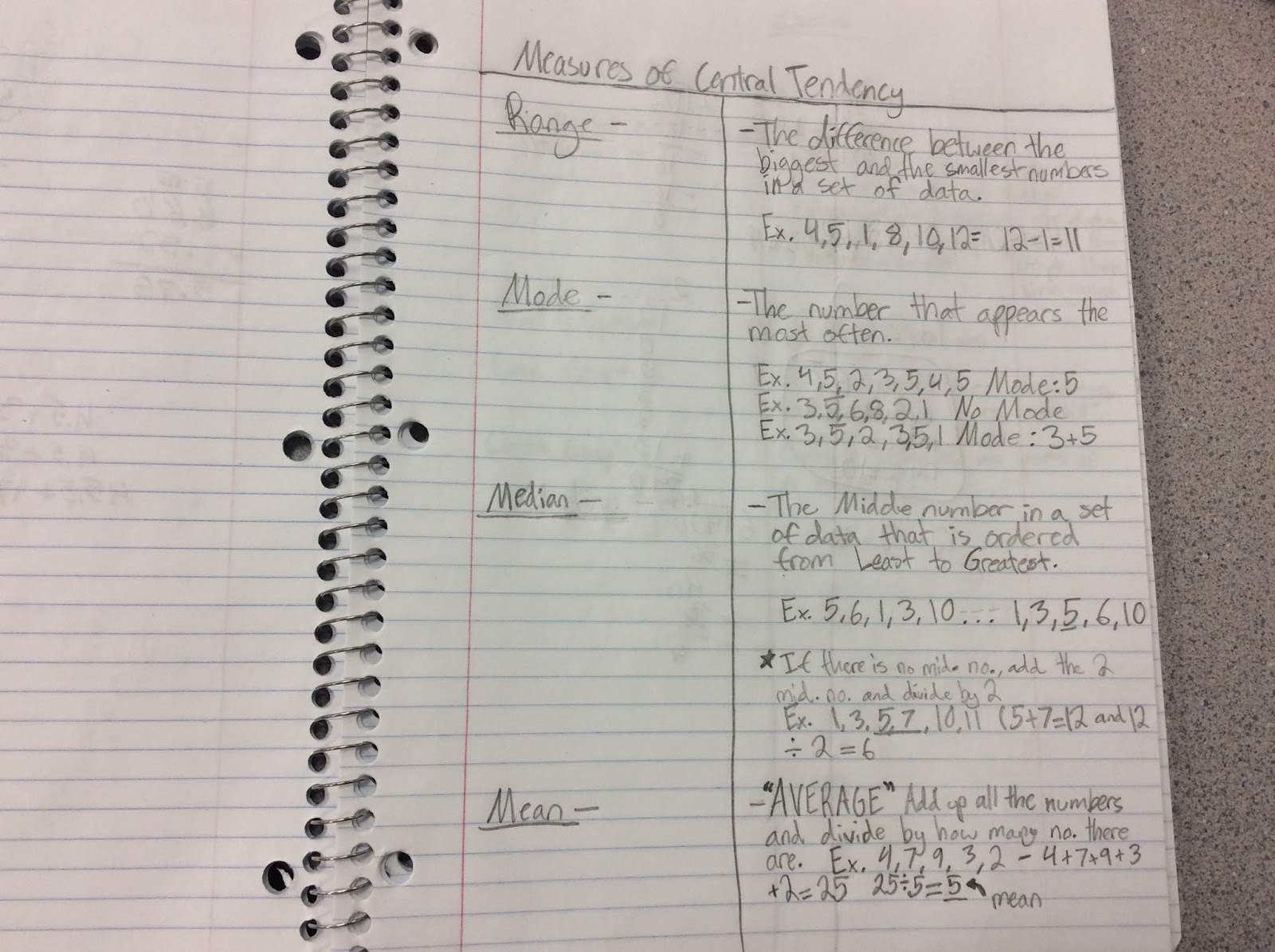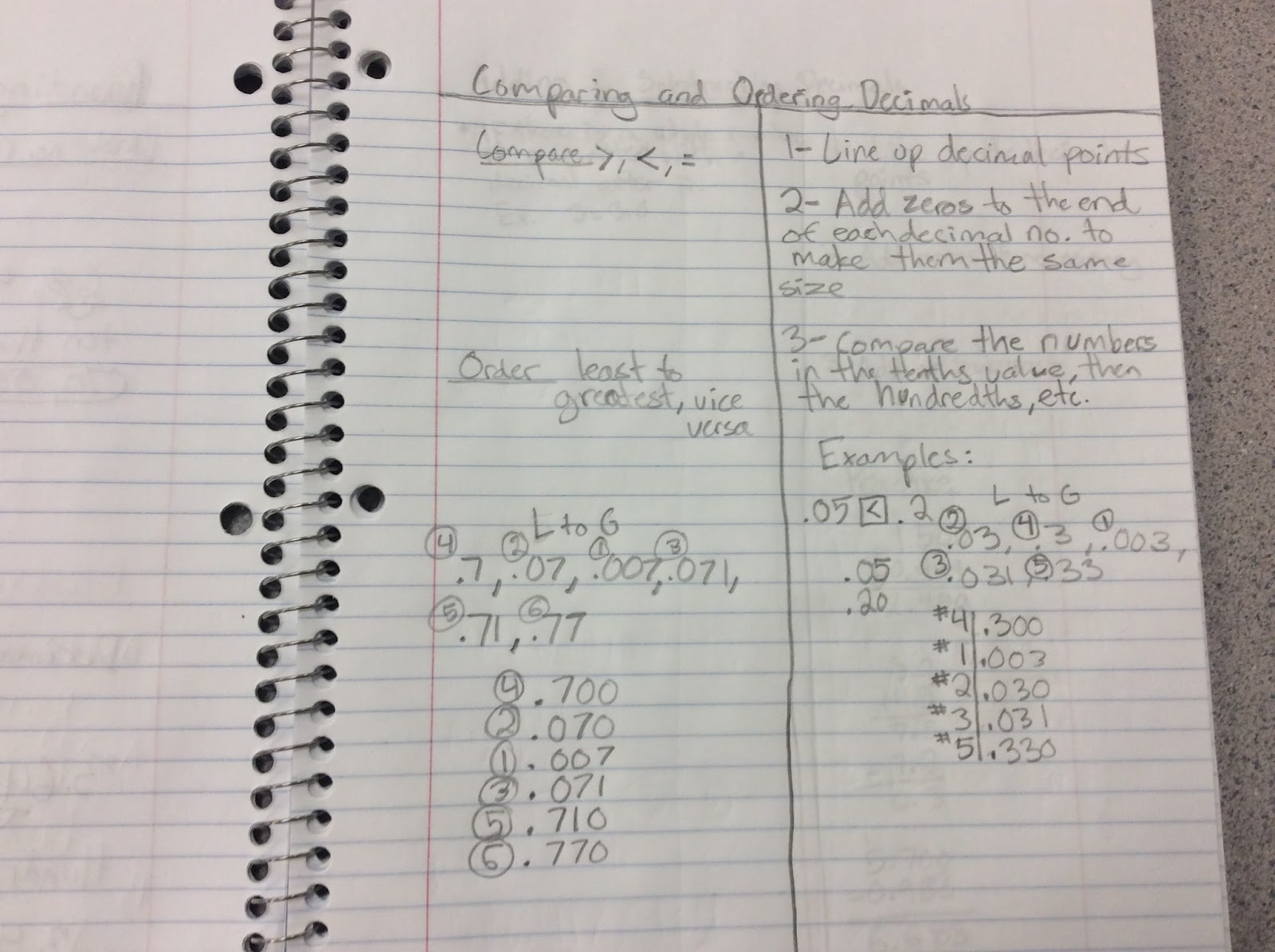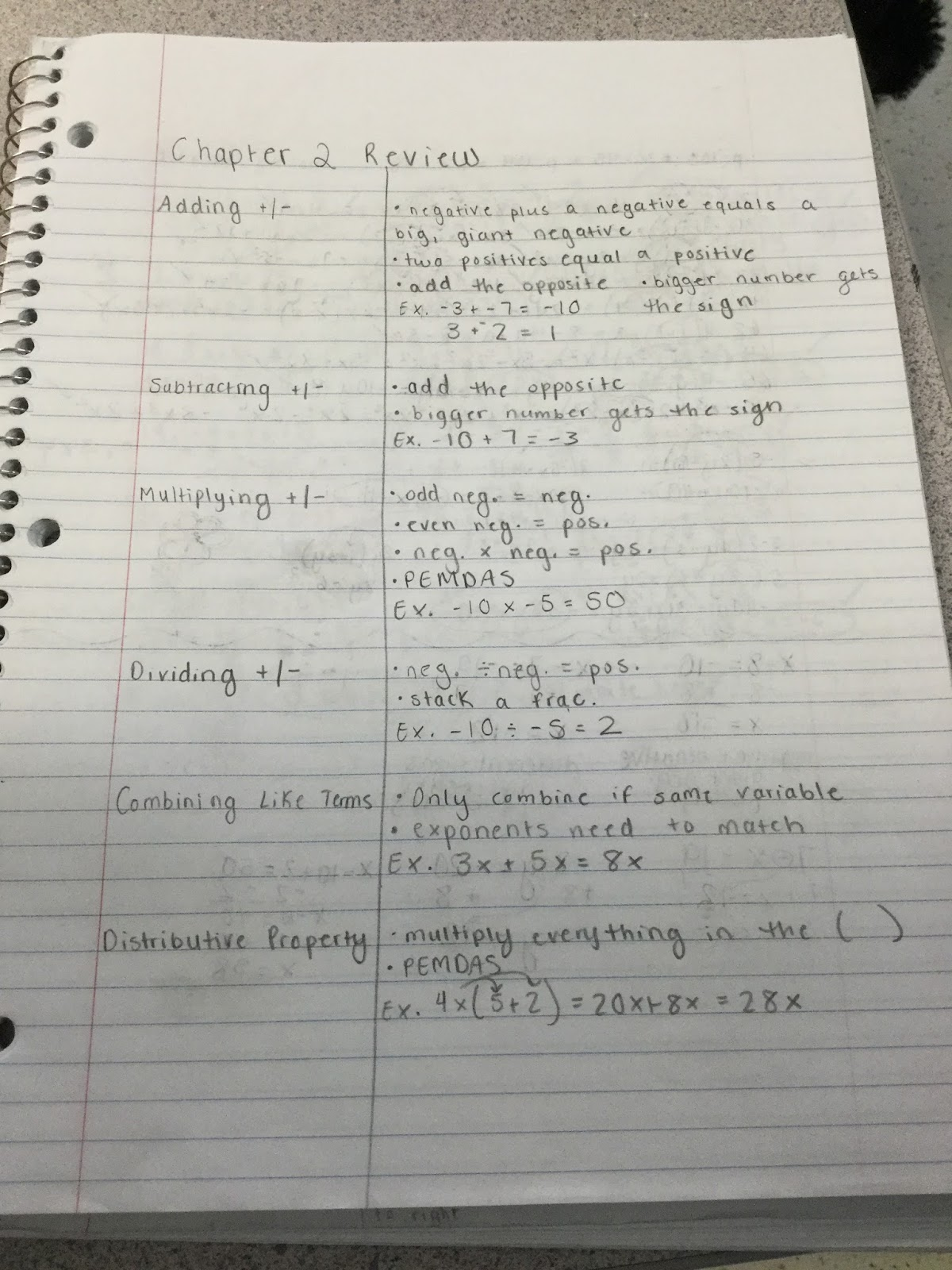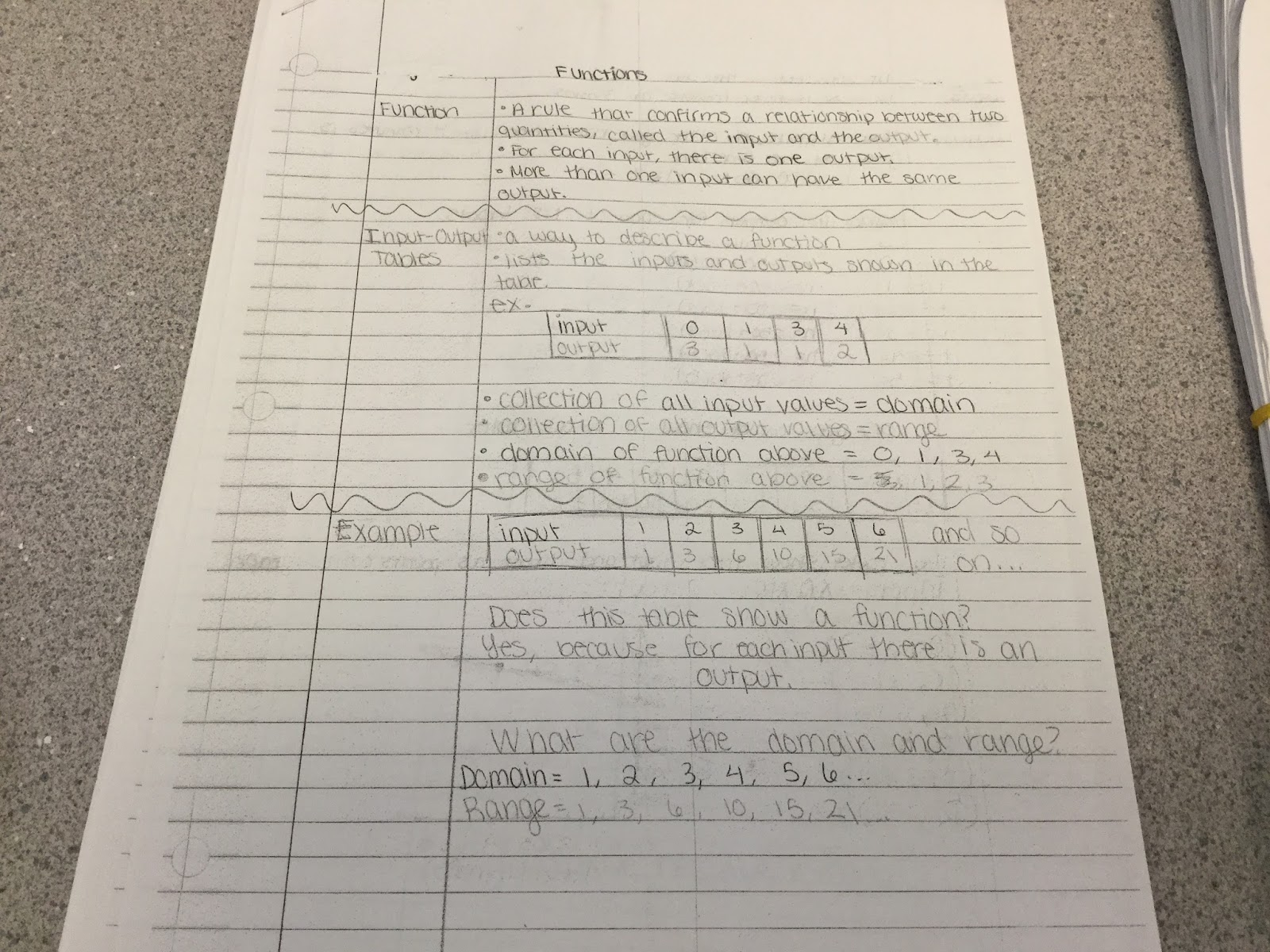## Friday, January 27, 2017

### Two-Column Notes in Math

In our math classes we use two-column notes for three different purposes. The first, and the one we use most often, is a two-column notes “101.”  We, the teachers, give the notes to the students for the them to copy down in their math notebooks.  The notes are either a mathematical process that they need to know, for example, how to compare and order decimals or how to graph the slope of a line.  Or the notes are for math vocabulary that the students need to know in order to perform the process they are learning. Here are a couple of examples.Two-column notes can also serve as a study guide for a quiz or test.  We begin the lesson by brainstorming the main topics from the chapter as a class and writing the topics on the board.  Students then work with partners to write the 2-column notes.  Partners write the main ideas from the class brainstorm in the left column and work together to generate appropriate details for the right column.  In math, these details may include rules, steps, and sample problems for each topic.  At the end of the lesson, we discuss the student-generated 2-column notes as a class.  We remind students to check their own notes and make additions and changes as needed based on class discussion. Here is an example of this.The third way students generate two column-notes is as part of a homework assignment.  We would assign “Read Chapter 3 Unit 1 and write 2-column notes for the main topics.”  We had mixed results the first time we did this.  Some of the notes were exemplary and even color-coded; others were too detailed; others were a total of four lines long.  One of the students asked, “Will you please show us how you would do this before you assign this again?”  This sounded like a valid request.  Rather than wait until next time, we read the section aloud with the students and  essentially did a think aloud of how we would generate the notes based on my reading.  We discussed what would serve as clues for main topics and students suggested “the bold vocab words,” “the headings on the pages,” “the parts labeled Example.”  Sometimes other students would guide the answers and sometimes we would.  Specifically, students agreed that bold vocab words as main topics were a good idea and “the parts labeled Example” were probably better served in the details section of the notes. Here is an example.Overall, we have had great success using two-column notes in our math classes.  As you can see, we use them for different purposes but each method (note taking, vocabulary, study guide, homework) is beneficial in its own way.  Two column notes have helped our students with organization, remembering material and gaining a deeper understanding of mathematics.

Cheryl Mantia and Kristin MacCurtain
MSMS Math Teachers